# GSEB Solutions Class 11 Physics Chapter 4 Motion in a Plane

Gujarat Board GSEB Textbook Solutions Class 11 Physics Chapter 4 Motion in a Plane Textbook Questions and Answers.

## Gujarat Board Textbook Solutions Class 11 Physics Chapter 4 Motion in a Plane

### GSEB Class 11 Physics Motion in a Plane Text Book Questions and Answers

Question 1.
State for each of the following physical quantities, if it is a scalar or a vector:
volume, mass, speed, acceleration, density, number of moles, velocity, angular frequency, displacement, angular velocity.
Scalar quantities: Volume, mass, speed, density, number of moles, angular frequency.
Vector quantities: Acceleration, velocity, displacement, angular velocity.

Question 2.
Pick out the two scalar quantities in the following list:
Force, angular momentum, work, current, linear momentum, electric field, average velocity, magnetic moment, reaction as per Newton’s third law, relative velocity?
Work, current.Question 3.
Pick out the only vector quantity in the following list:
Temperature, pressure, impulse, time, power, total path, length, energy, gravitational potential, coefficient of friction, charge.
Impulse. As impulse = Force × time = change in momentum.
As force and momentum are vector quantities, so it is.

Question 4.
State, with reasons, whether the following algebraic operations with scalar and vector physical quantities are meaningful:

2. adding a scalar to a vector of the same dimensions,
3. multiplying any vector by any scalar,
4. multiplying and two scalars,
6. adding a component of a vector to the same vector.

1. No, adding any two scalars is not meaningful because only the scalars of same dimensions (i.e. of same nature) can be added.

2. No, adding a scalar to a vector of the same dimension is not meaningful because a scalar cannbt be added to a vector.

3. Yes, multiplying any vector by any scalar is meaningful algebraic operation. It is because when any vector is multiplied by any scalar, then we get a vector having magnitude equal to scalar number times the magnitude of the given vector, e.g. when acceleration a is multiplied by mass m, we get force F = ma which is a meaningful operation.

4. Yes, the product of two scalars gives a meaningful result e.g. when power P is multiplies by time t, then we get work done (W) i.e. W = Pt, which is a useful algebraic operation.

5. No, as the two vectors of same dimensions (i.e. of the same nature) can only be added, so addition of any two vectors is not a meaningful algebraic operation.

6. Yes, a component of a vector can be added to the same vector as both of them are the vectors of same nature i.e. same dimensions, e.g. component of velocity can be added to the velocity. So only (c) and (d) are meaningful operations.Question 5.
Read each statement below carefully and state with reasons if it is true or false?

1. The magnitude of a vector is always1 a scalar.
2. Each component of a vector is always a scalar.
3. The total path length is always equal to the magnitude of the displacement vector of a particle.
4. The average speed of a particle, defined as total path length divided by the time taken to cover the path, is either greater or equal to the magnitude of average velocity of the particle over the same interval of time.
5. Three vectors not lying in a plane can never add up to give a null vector.

1. True; because magnitude of a vector is a pure number.

2. False; as each component of a given vector is always a vector.

3. True; only if the particle moves along a straight line in the same direction otherwise false.

4. True; because the total path length is either greater than or equal to the magnitude of the displacement vector, so the average speed is greater or equal to the magnitude of average velocity.

5. True; as they cannot be represented by the three sides of a triangle taken in the same order. Here the resultant of any two vectors will be in the plane of these two vectors only and it cannot balance the third vector which is in a different plane. Two vectors, can cancel each other’s effect only if they are equal in magnitude and opposite in direction.

Question 6.
Establish the following vector inequalities geometrically or otherwise:
(a) |a + b| < |a| + |b|
(b) |a + b| ≥ |a| – |b|
(c) |a – b| ≤ |a| + |b|
(d) |a – b| ≥ |a| – |b|
When does the equality sign above apply?
Let the two vectors $$\vec{a}$$ and $$\vec{b}$$ be represented by the sides OP and PQ of the ∆OPQ taken in the same order, then their resultant is represented by the side OQ of the ∆ such that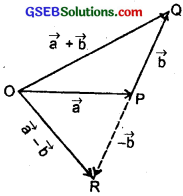OQ = a + b as shown in fig.
Thus OP = a, PQ = b
∴ |OP| = |a|, |PQ| = |b|
and |OQ| = |a + b|

(a) |a + b| ≤ |a| + |b|

Proof: We know from the property of a triangle that its one side is always less than the sum of the lengths of its two other sides.
Now in ∆OPQ,
OQ < OP + PQ
or |a + b| < |a| + |b| …………………. (i) If the two vectors a and b are acting along the same straight line i.e. are collinear and in the same direction, then θ(angle between them) = 0 ∴ |a + b| = $$\sqrt{a^{2}+b^{2}+2 a b \cos \theta}$$ = $$\sqrt{(a+b)^{2}}$$ = a + b = |a| + |b| ………………….. (ii) Combining (i) and (ii), we get |a + b| ≤ |a| + |b| The equality sign applies if a and b are collinear and act in same direction.

(b) |a + b| ≥ ||a| + |b|| Proof: One side is more than the difference of two the other sides i.e. in ∆OPQ in fig. OQ > |OP – PQ|
The modulus of (OP – PQ) has been taken because L.H.S. is +ve and R.H.S. may be -ve if OP < PQ. or |a + b| > ||a| – |b|| …………………… (iv)
If a and b are acting along the same straight line in the opposite direction, then
|a + b| = ||a| – |b|| …………………….. (v)
combining (iii) and (iv), we get
|a + b| ≥ ||a| – |b||
Equality sign holds for as stated in eqn. (iv)

(c) |a – b| ≤ |a| + |b|
Proof: Again in AOPR in fig.
OP = |a|, PR = |-b| = b
OR = |a – b|
As one side of a triangle is less than the sum of the other two sides,
∴ OR < OP + PR
or |a – b| < |a| + |-b| < |a| + |b| ……………. (v) Also if the two vectors a and b are acting along a straight line in the opposite direction, then |a – b| = |a| + |b| ………………….. (vi) Combining (v) and (vi), we get |a – b| ≤ |a| + |b| Hence proved.

(d) |a – b| ≥ ||a| – |b|| As one side of a triangle is more than the difference of its two other sides, thus in ∆OPR in fig. OR > |OP – PR|
The modulus of (OP – PR) has been taken because the L.H.S. is always positive but the R.H.S. may be negative if OP < PR, thus
|a – b| ≥ ||a – |b|| …………………. (vii) (∵ OR = |a – b|, OP = |a|, PR = |b|)
Also if the two vectors a and b are acting along the same straight line in the same direction, then
|a – b| = ||a| – |b|| …………………….. (viii)
Combining (vii) and (viii), we get
|a – b| ≥ ||a| – |b||
Hence proved.Question 7.
Given a + b + c + d = 0, which of the following statements are correct:
(a) a, b, c and d must each be a null vector.
(b) The magnitude of (a + c) equals the magnitude of (b + d).
(c) The magnitude of a can never be greater than the sum of the magnitudes of b, c and d.
(d) b + c must lie in the plane of a and d if a and d are not collinear, and in the line of a and d, if they are collinear?
(a) Not correct, because a + b + c + d can be zero in many ways other then a, b, c and d must each be a null vector.

(b) correct, as a + b + c + d = 0
∴ a + c = – (b + d)
or |a + c| = |b + d|

(c) correct, as a + b + c + d
∴ a = -(b + c + d)
or |a| = |b + c +d| …………………(i)
From equation (i), we see that a magnitude of a is equal to the magnitude of vector (b + c + d). Since the sum of the magnitudes of b, c, d my be equal or greater than the magnitude of a, hence the magnitude of a can never be greater than the sum of the magnitudes of b, c and d.

(d) correct:
As a + b + c + d = 0
or a + (b + c) + d = 0
The resultant sum of the three vectors a, (b + c) and d can be zero only if (b + c) lies in the plane of a and d if a and d are not collinear and these three vectors are represented by the three sides of a triangle taken in the same order. If a and d are collinear, then (b + c) must be in the line of a and d, only then the vector sum of all the vectors will be zero.Question 8.
Three girls skating on a circular ice ground of radius 200 m start from a point P on the edge of the ground and reach a point Q diametrically opposite to P following different paths as shown. What is the magnitude of the displacement vector for each? For which girl is this equal to the actual length of path skated?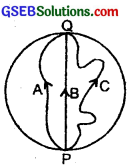Let the three girls be A, B and C. Let PAQ, PBQ and PCQ be the paths followed by A, B and C respectively. Radius of the circular track = 200 m.
As all the girls start from point P and reach at Q.
∴ Displacement vector for each girl = $$\overrightarrow{\mathrm{PQ}}$$
so the magnitude of the displacement vector for each girl
= $$\overrightarrow{\mathrm{PQ}}$$
= Diameter of the circular ice ground
= 2 × 200
= 400 m.
From Fig. 4.2 it is clear that for girl B, the magnitude of the displacement vector is equal to the actual length of the path skated.

Question 9.
A cyclist starts from the centre O of a circular park of radius 1 km, reaches the edge P of the park, then cycles along the circumference, and returns to the centre along QO as shown. If the round trip takes 10 min, what is the (a) net displacement, (b) average velocity and (c) average speed of the cyclist?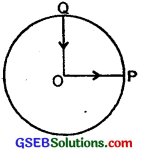(a) Radius of circular track = 1 km
As both the initial and final positions of the cyclist are the same, so the net displacement of the cyclist is zero.
(b) Average velocity of the cyclist =Since the net displacement of the cyclist is zero, therefore the average velocity of cyclist is = $$\frac{0}{total taken}$$ = 0

(c) We know that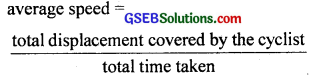…………………… (i)
Now total distance covered = Total path length
= OP + Actual distance PQ + QO
= 1 km + $$\frac{1}{4}$$ × 2πr + 1 km
= 1 km + $$\frac{2π×1}{4}$$ + 1 km (here r = 1 km)
= 1 + $$\frac{22}{7}$$ × $$\frac{1}{2}$$ + 1 = $$\frac{25}{7}$$ km …………………… (ii)
Total time taken = 10 minutes = $$\frac{1}{6}$$ hour ……………………… (iii)
∴ from (i), (ii) and (iii), we get
average speed = $$\frac{25/7}{1/6}$$
= $$\frac{25}{7}$$ × 6 = $$\frac{150}{7}$$ = 21.43 kmh-1.Question 10.
On an open ground, a motorist follows a track that turns to his left by an angle of 60° after every 500 m. Starting from a given turn, specify the displacement of the motorist at the third, sixth and eighth turn. Compare the magnitude of the displacement with the total path length covered by the motorist in each case?
Here, the path followed by the motorist is a closed regular hexagonal path ABCDEF of side length 500 m as shown in figure. Let the motorist starts his journey from point A, then he reaches D at the end of third turn, at A at the end of 6th turn and at point C at the end of 8th turn.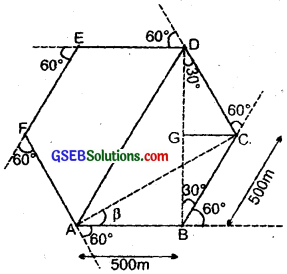(i) Third turn: He takes the third turn at point D.
∴ Displacement vector at D = $$\vec {AD}$$ = $$\vec {AB}$$ + $$\vec {BC}$$ + $$\vec {CD}$$
Calculation of the magnitude of $$\vec {AD}$$:
join BD, Now Draw CG perpendicular on BD, so BG = GD BG
From ∆BCG, $$\frac{BG}{BC}$$ = cos 30°
∴ BG = BC cos 30° = BC × $$\frac{\sqrt{3}}{2}$$
= 500 × $$\frac{\sqrt{3}}{2}$$ = 250$$\sqrt{3}$$m
Similarly from rt. $$\frac{DG}{DC}$$ = cos 30° or DG = 250$$\sqrt{3}$$ m
∴ BD = BG + GD = 250$$\sqrt{3}$$ + 250$$\sqrt{3}$$
= 500$$\sqrt{3}$$
Now from rt. AD2 = AB2 + BD2
= (500)2 + (500$$\sqrt{3}$$)2
= (500)2 (1 + 3) = (2 × 500)2
∴ AD = 1000 m = 1 km.
Thus displacement of the motorist at the end of 3rd turn is 1000 m in the direction of $$\vec{AD}$$ i.e. at 60°.
But total path length from A to D = AB + BC + CD
= 500 + 500 + 500 = 1500 m = 1.5 km

(ii) Sixth turn:
As the motorist is at the starting point i.e. A at end of sixth turn, hence his displacement vector = null vector whereas total path length
= AB + BC + CD + DE + EF + FA
= 6 × 500 = 3000 m
= 3 km.

(iii) Eighth turn:
He takes 8th turn at point C,
∴ Displacement vector of the motorist = $$\vec {AC}$$
Thus using ∆ law of vector addition, the magnitude of $$\vec {AC}$$ is given by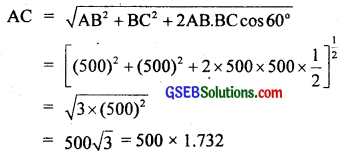= 866 m
Let the direction at 8th turn at <β w.r.t. initial direction i.e. $$\vec {AB}$$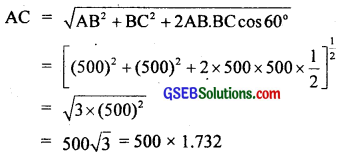∴ β = 30°
Thus the displacement of the motorist at the 8th turn is 866 m making an angle of 30° with the initial direction.
Total path length = 8 × 500 = 4000 m = 4km.
Comparison of the magnitude of displacement with the total path length in each case: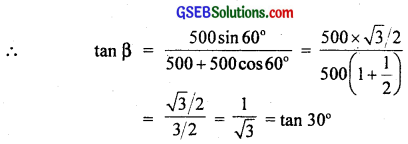Question 11.
A passenger arriving in a new town wishes to go from the station to a hotel located 10 km away on a straight road from the station. A dishonest cabman takes him along a circuitoius path 23 km long and reaches the hotel in 28 min. What is (a) the average speed of the taxi (b) the magnitude of average velocity? Are the two equal?
Magnitude of the displacement = 10 km
Distance covered = 23 km
Time taken = 28 min = $$\frac{28}{60}$$ = $$\frac{7}{15}$$ h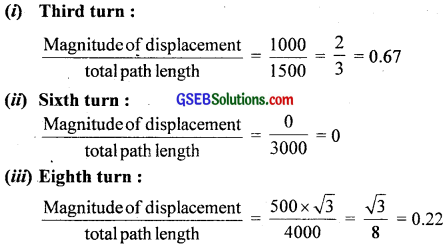= 49.3 kmh-1.

(b)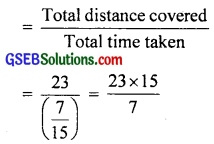= 21.43 kmh-1
Clearly, the average speed and the magnitude of average velocity are not equal. They are equal only for a straight path.

Question 12.
Rain is falling vertically with a speed of 30 ms-1. A woman rides a bicycle with a speed of 10 ms-1 in the north to south direction. What is the direction in which she should hold her umbrella?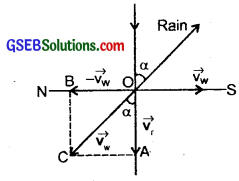Let the rain be falling vertically downward with the speed of 30 ms-1 along OA. The woman is moving along OS with a speed of 10 ms-1 To protect herself from rain, the woman should hold her umbrella in the direction of relative velocity of the rain with respect to the woman i.e. vrw. To determine the vrw, the woman must be brought to rest by imposing an equal and opposite velocity of the observer both on woman as well as on the rain. Rain thus has two velocities:

1. Its own velocity of 30 ms-1 along $$\overrightarrow{\mathrm{OA}}$$.
2. Imposed velocity, 10 ms-1 along $$\overrightarrow{\mathrm{OB}}$$

The relative velocity is the resultant of these two velocities.
i.e. Vrw = Vr + (-Vw)
∴ |Vrw| = $$\sqrt{30^{2}+10^{2}}=10 \sqrt{10}$$ ms-1
Let α = angle made by the relative velocity with the vertical, then in ∆OCA,
tan α = $$\frac{AC}{OA}$$ = $$\frac{OB}{OA}$$ = $$\frac{10}{30}$$
= $$\frac{1}{3}$$ = 0.3333
Tan α = tan 18°26′
∴ α = 18°26′
So woman should hold umbrella at an angle of 18°26′ with the vertical towards south.Question 13.
A man can swim with a speed of 4 kmh-1 in still water. How long does he take to cross a river 1 km wide if the river flows steadily 3 kmh-1 and he makes his strokes normal to the river current. How far down the river does he go when he reaches the other bank?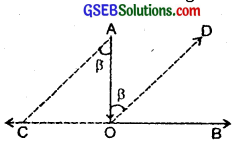Speed of man, Vs = 4 kmh-1
Distance travelled = 1 km
Speed of river = 3 kmh-1
If t = time taken to cross the river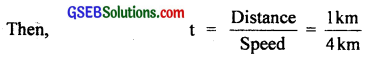= $$\frac{1}{4}$$ hour = 15 minutes
The man is carried down by stream with velocity of river water.
∴ Distance travelled by man in 15′ (or $$\frac{1}{4}$$h) is
= 3 kmh-1 × $$\frac{1}{4}$$h
= 750 m.

Question 14.
In a harbour, wind is blowing at the speed of 72 kmh-1 and the flag on the mast of a boat anchored in the harbour flutters along N-E direction. If the boat starts moving at the speed of 51 kmh-1 of the north, what is the direction of the flag on the mast of the boat?
Let Vw = velocity of wind along NE direction.
Vb = velocity of boat along N direction = 51 kmh-1 along north.
When the boat is anchored in the harbour, the flag flutters along the NE direction, so the velocity of the wind is along NE direction. When the boat starts moving along North direction, the flag will flutter along the direction of relative velocity of wind with respect to the boat.
Here Vw = 30 kmh-1 along NE
Vb = 51 kmh-1 along N
Thus if Vwb = relative velocity of wind w.r.t. boat, Vwb can be determined by bringing the boat to rest by imposing an equal and opposite velocity on boat as well as on the wind. Thus wind has two velocities.

1. Its own velocity, Vw = 30 kmh-1 along NE direction.
2. Imposed velocity, Vb = 51 kmh-1 along S direction.

i.e. thus Vwb is the resultant of these two velocities i.e.
Vwb = Vw + (-Vb)
It is clear from the figure that angle between Vw and – Vb is 45° + 90° = 135°.
If β be the angle made by Vwb with Vw, then according to the parallelogram law of vector addition, the direction of flutter of flag is given by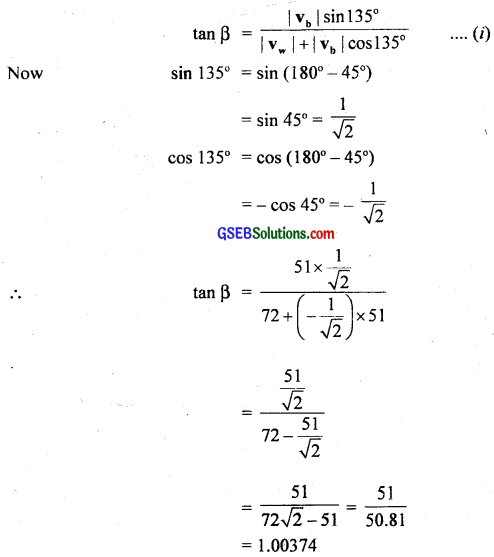Now sin 135° = sin (180° – 45°)
= sin 135° = sin (180° – 45°)
= sin 45° = $$\frac{1}{\sqrt{2}}$$
cos 135° = cos(180° – 45°)
= – cos 45° = – $$\frac{1}{\sqrt{2}}$$= 1.00374
or tan β = tan 45°6′
∴ β = 45°6′ = 45.1° w.r.t. NE direction
Thus the flag will flutter at 45.1° w.r.t NE direction i.e. direction of wind blow
or (45.1°- 45°) = 0.1° w.r.t. east direction i.e. almost due east.Question 15.
The ceiling of a long hall is 25 m high. What is the maximum horizontal distance that a ball thrown with a speed of 40 ms-1 can go without hitting the ceiling of the hall?
Initial velocity i.e. velocity of projection of ball, u = 40 ms-1.
The maximum height attained by the ball is given by
H = $$\frac{\mathrm{u}^{2} \sin ^{2} \theta}{2 \mathrm{~g}}$$ …………………… (1)
Here, H = 25 m, u = 40 ms-1, g = 9.8 ms-2 ………………………… (2)
∴ from equation (1) and (2), we get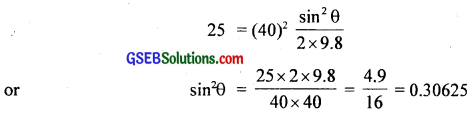∴ sin θ = $$\sqrt{0.3063}$$ = 0.5534
= sin 33°36′
∴ 2θ = 66°72′ = 67°12′
∴ Maximum Horizontal Range, R = ?
We know that the formula for R is given by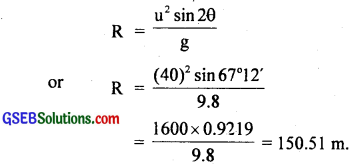Aliter: sin2θ = 0.3063 (as above)
Also cos θ = $$\sqrt{1-\sin ^{2} \theta}$$ = $$\sqrt{1-0.3063}$$
= $$\sqrt{.6937}$$ = 0.8329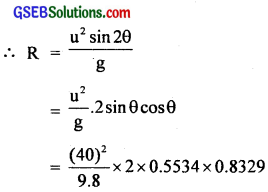= 150.50 m.

Question 16.
A cricketer can throw a ball to a maximum horizontal distance of 100 m. How much high above the ground can the cricketer throw the same ball?
We know the horizontal range is given by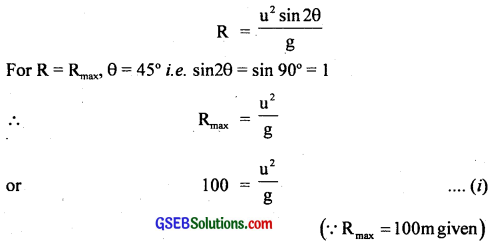Let H = height upto which the ball goes when the cricketer throws it with velocity u. Since the final velocity of the ball, v = 0.
∴ Using the relation,
v2 – u2 = 2as, we get
02 – u2 = 2 (-g) H (∵ here, v = 0, a = -g, s = H)
or H = $$\frac{\mathrm{u}^{2}}{2 \mathrm{~g}}$$
or H = $$\frac{1}{2}$$ ($$\frac{\mathrm{u}^{2}}{\mathrm{~g}}$$)
= $$\frac{1}{2}$$ × 100 [by using (i)]
∴ H = 50 m.Question 17.
A stone tied to the end of a string 80 cm long is whirled in a horizontal circle with a constant speed. If the stone makes 14 revolutions in 25 s, what is the magnitude and direction of acceleration of the stone?
Radius of the horizontal circle, r = 80 cm = 0.80
n = 14
t = 25″
∴ ν = $$\frac{n}{t}$$
Angular speed of revolution of the stone,
ω = $$\frac{θ}{t}$$ = $$\frac{2πn}{t}$$ = 2π($$\frac{n}{t}$$)
= 2 × $$\frac{22}{7}$$ × ($$\frac{14}{25}$$)
= $$\frac{88}{25}$$ rads-1
∴ Magnitude of acceleration produced in the stone, will be equal to the magnitude of centrepetal acceleration
= rω2
= 0.80 × ($$\frac{88}{25}$$)2
= 0.80 × $$\frac{88}{25}$$ × $$\frac{88}{25}$$ = 9.91ms-2
The direction of the acceleration is towards the centre of the circle along its radius at every point.

Aliter:
r = 80 cm = 0.80 m
ν = $$\frac{14}{25}$$ rev s-1
∴ ω = 2πν = 2 × $$\frac{22}{7}$$ × ($$\frac{14}{25}$$)
= $$\frac{88}{25}$$ rev/s
a = rω2 = 9.91 ms-1

Question 18.
An aircraft executes a horizontal loop of radius 1 km with a speed of 900 kmh-1. Compare its centrepetal acceleration with the acceleration due to gravity?
Here, r = 1 km = 1000 m;
v = 900 kmh-1 = 900 × $$\frac{1000}{3600}$$ms-1
= 250 ms-1
The centrepetal acceleration of the aircraft is given by
a = $$\frac{v^{2}}{r}=\frac{(250)^{2}}{1000}$$
= $$\frac{62500}{1000}$$ = 62.5 ms-2
Acceleration due to gravity,
g = 9.8 ms-2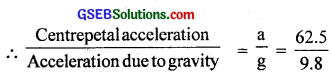or $$\frac{a}{g}$$ = 6.4
or a = 6.4 g.

Question 19.
Read each statement below carefully and state with reasons, if it is true or false:
(a) The net acceleration of a particle in the circular motion is always along the radius of the circle towards the centre.
(b) The velocity vector of a particle at a point is always along the tangent to the path of the particle at that point.
(c) The acceleration vector of a particle in uniform circular motion averaged over one cycle is a null vector.
(a) The statement is false since the centripetal acceleration is towards the centre only in the case of uniform circular motion (constant speed) for which its is true.

(b) True, the velocity of a particle is always along the tangent to the path of the particle at the point either in rectilinear or circular or curvilinear motion.

(c) True, because the direction of acceleration vector is always changing with time, always being directed towards the centre of the path followed in the uniform circular motion, so the resultant of all these vectors will be a null vector.Question 20.
The position of a particle is given by
$$\vec {r}$$ = 3.0t$$\hat {i}$$ – 2.0t$$\hat {j}$$ + 4.0$$\hat {k}$$m
where t is in seconds and the coefficients have the proper units for r to be in meters.
(a) Find the $$\vec {V}$$ and $$\vec {a}$$ of the particle?
(b) What is the magnitude and direction of velocity of the particle at t = 2s?
The position vector (r) of the particle is
r = 3.0t$$\hat {i}$$ – 2.0t2$$\hat {j}$$ + 4.0 $$\hat {k}$$m …………………. (i)
(a) We know the velocity v(t) of the particle is given by
V(t) = $$\frac{dr}{dt}$$ = $$\frac{d}{dt}$$(r)
= $$\frac{d}{dt}$$(3.0t$$\hat {i}$$ – 2.0t2$$\hat {j}$$ + 4.0 $$\hat {k}$$)
= 3$$\hat {i}$$ – 4t$$\hat {j}$$ + 0 ……………………… (ii)
Also we know that acceleration a(t) of the particle is given by
a(t) = $$\frac{dv(t)}{dt}$$ = $$\frac{d}{dt}$$ v(t)
= $$\frac{d}{dt}$$ (3$$\hat {i}$$ – 4t$$\hat {j}$$) [by using (ii)]
= 0 – 4$$\hat {j}$$
∴ a(t) = -4$$\hat {j}$$ ………………….. (iii)

(b) We know that at time t, the velocity of the particle is given by according to equation (ii)
v(t) = 3$$\hat {i}$$ – 4t$$\hat {j}$$
∴ At t = 2s,
V = 3.0$$\hat {i}$$ – 4

= $$\frac{d}{dt}$$ (3$$\hat {i}$$ – 4t$$\hat {j}$$) [by using (ii)]
v(t) = 3$$\hat {i}$$ – 4t$$\hat {j}$$
∴ At t = 2s,
v = 3.0$$\hat {i}$$ – 4 × 2$$\hat {j}$$
= 3.0$$\hat {i}$$ – 8$$\hat {j}$$
∴ Its magnitude is
v = $$\sqrt{3^{2}+(-8)^{2}}$$ = $$\sqrt{9+64}$$
= $$\sqrt{73}$$ = 8.544 ms-1
and direction of ν is given by
θ = tan-1 ($$\frac{\mathbf{v}_{\mathrm{y}}}{\mathrm{V}_{\mathrm{x}}}$$) = tan-1 (-$$\frac{8}{3}$$)
= 70° with x-axis.

Question 21.
A particle starts from the origin at t = 0s with a velocity of 10.0 $$\hat {j}$$ m/s and moves in the x – y plane with the constant acceleration of (8.0$$\hat {i}$$ + 2.0$$\hat {j}$$) ms-2.
(a) At what time is the x-coordinate of the particle 16 m? What is the y-coordinate of the particle at that time?
(b) What is the speed of the particle at the time?
Here, u0 (at t = 0) = 10.0 $$\hat {j}$$ ms-1
acceleration, a = (8.0$$\hat {i}$$ + 2.0$$\hat {j}$$) ms-2

(a) We know that the position is given by
r(t) = u0.t + $$\frac{1}{2}$$at2
= 10.0$$\hat {j}$$.t + $$\frac{1}{2}$$(8.0$$\hat {i}$$ + 2.0$$\hat {j}$$)t2
= 4.0t2$$\hat {i}$$ + (10.0t + 1.0t2)$$\hat {j}$$ …………………… (i)
∴ in equation (i), the x and y coordinates of the particle are given by
x(t) = 4.0t2 ……………………. (ii)
and y(t) = 10.ot + 1.0t2 ……………………. (iii)
But x(t) = 16 m(given in the statement) ……………………. (iv)
∴ From (ii) and (iv), we get
16 = 4.0 t2
or t2 = 16/4 = 4 = 22
∴ t = 2 sec.
Putting t = 2s in equation (iii), we get the y-coordinate as follows:
y(t) = 10.0t = 1.0t2
= 10.0 × 2 + 1.0 × 22
= (20 + 4.0) = 24 m

(b) Speed of the particle:
We know that velocity of the particle is given by
V = $$\frac{d}{dt}$$(r)
or V = $$\frac{d}{dt}$$[4.0 t2$$\hat {i}$$ + (10.0t + 1.0t2)$$\hat {j}$$]
= 4.0 × 2t$$\hat {i}$$ + (10.0t + 2t)$$\hat {j}$$
= 8t$$\hat {i}$$ + (10.0 + 2t)$$\hat {j}$$,
∴ As t = 2s v(t) = 8 × 2$$\hat {i}$$ + (10.0 + 2 × 2)$$\hat {j}$$
or v(t) = 16$$\hat {i}$$ + 14$$\hat {j}$$
∴ Speed of the particle at t = 2s is given by
|v| = $$\sqrt{v_{x}^{2}+v_{y}^{2}}=\sqrt{16^{2}+14^{2}}$$
= $$\sqrt{256+196}$$ = $$\sqrt{452}$$
= 21.26 ms-1.Question 22.
$$\hat {i}$$ and $$\hat {j}$$ are unit vectors along x and y axis respectively. What is the magnitude and direction of vectors $$\hat {i}$$ + $$\hat {j}$$ and $$\hat {i}$$ – $$\hat {j}$$? What are the components of a vector A = 2$$\hat {i}$$ + 3$$\hat {j}$$ along the direction $$\hat {i}$$ + $$\hat {j}$$ and $$\hat {i}$$ – $$\hat {j}$$? (You may use graphical method).(i) Magnitudes of ($$\hat {i}$$ + $$\hat {j}$$) and ($$\hat {i}$$ – $$\hat {j}$$) vectors: As $$\hat {i}$$ and $$\hat {j}$$ are the unit vectors acting along X and Y axis respectively.
The magnitude of vector $$\hat {i}$$ + $$\hat {j}$$ is given by
|$$\hat {i}$$ + $$\hat {j}$$| = $$\sqrt{1^{2}+1^{2}+2.1 .1 \cdot \cos 90^{\circ}}$$
= $$\sqrt{2+0}$$
= $$\sqrt{2}$$ = 1.414 units
The magnitude of vector $$\hat {i}$$ – $$\hat {j}$$ is given by
|$$\hat {i}$$ – $$\hat {j}$$| = $$\sqrt{1^{2}+1^{2}-2.1 .1 \cdot \cos 90^{\circ}}$$
= $$\sqrt{2}$$ = 1.414 units

(ii) Directions of $$\hat {i}$$ + $$\hat {j}$$ and $$\hat {i}$$ – $$\hat {j}$$:
Let θ be the angle made by $$\hat {i}$$ + $$\hat {j}$$ with $$\hat {i}$$ i. e. with X – axis.
∴ By definition of scalar product of tvvo vectors
($$\hat {i}$$ + $$\hat {j}$$).$$\hat {i}$$ = |$$\hat {i}$$ + $$\hat {j}$$| |$$\hat {i}$$| cosθ
or $$\hat {i}$$. $$\hat {i}$$ + $$\hat {i}$$. $$\hat {j}$$ = $$\sqrt{2}$$ × 1 × cosθ
or 1 + 0 = $$\sqrt{2}$$ cosθ (∵ $$\hat {i}$$.$$\hat {i}$$ = 1 and $$\hat {i}$$.$$\hat {j}$$ = 0)
or cos θ = $$\frac{1}{\sqrt{2}}$$ = cos 45°
∴ θ = 45°
Also let α be the angle made by $$\hat {i}$$ – $$\hat {j}$$ with $$\hat {i}$$ i.e. with X – axis
Then ($$\hat {i}$$ – $$\hat {j}$$).$$\hat {i}$$ = |$$\hat {i}$$ – $$\hat {j}$$| |$$\hat {i}$$| cos α
or $$\hat {i}$$.$$\hat {i}$$ – $$\hat {i}$$.$$\hat {j}$$ = $$\sqrt{2}$$ × 1 × cos α
or 1 – 0 = $$\sqrt{2}$$ cos α
or cos α = $$\frac{1}{\sqrt{2}}$$ = cos 45°
As $$\hat {j}$$ is -ve, so $$\hat {i}$$ – $$\hat {j}$$ makes – 45° with $$\hat {i}$$
Thus both ($$\hat {i}$$ + $$\hat {j}$$) and ($$\hat {i}$$ – $$\hat {j}$$) act at 45° and -45° with X-axis respectively as shown in figure.

(iii) (a) Let us now determine the component of A = 2$$\hat {i}$$ + 3$$\hat {j}$$ in the direction of ($$\hat {i}$$ + $$\hat {j}$$)
Let B = $$\hat {i}$$ + $$\hat {j}$$
∴ A.B = AB cos β = (A cos β)B
or A cos β = $$\frac{A.B}{B}$$
or magnitude of the component of A in the direction of B i.e ($$\hat {i}$$ + $$\hat {j}$$) is A cos β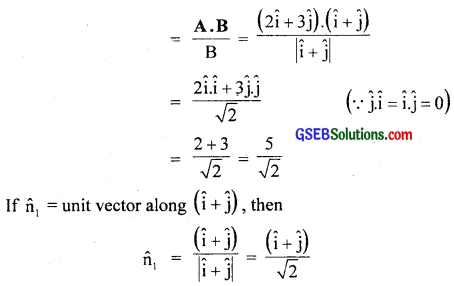∴ Component of A along ($$\hat {i}$$ + $$\hat {j}$$) = (Magnitude of the component of A along $$\hat {i}$$ + $$\hat {j}$$)$$\hat{\mathrm{n}}$$1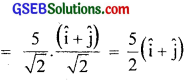(b) Let us now determine the component of A along $$\hat {i}$$ – $$\hat {j}$$:
Let $$\hat{\mathrm{n}}$$2 = unit vector acting along $$\hat {i}$$ – $$\hat {j}$$: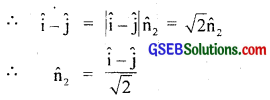∴ Magnitude of the component of A along ($$\hat {i}$$ – $$\hat {j}$$)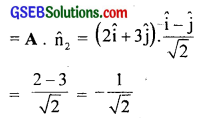∴ Component of A along ($$\hat {i}$$ – $$\hat {j}$$)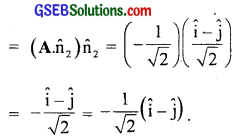Question 23.
For any arbitrary motion in space which of the following relations are true.
(a) Vaverage = $$\frac{1}{2}$$ [v(t1) + v(t2)]
(b) Vaverage = [r(t2) – r(t1)/(t2 – t2)]
(c) V(t) = V(0) + a t
(d) r(t) = r(0) _ + v(0)t + $$\frac{1}{2}$$ at2
(e) aaverage= [v(t2) – v(t1)]/(t2 – t1)
(The average stands for average of the quantity over the time interval t1 to t2).
Relations (b) and (e) are true for any arbitrary motion in space. Relations (a), (c) and (d) are false as they hold for uniformly accelerated motion. For arbitrary motion, acceleration is not uniform.

Question 24.
Read each statement below carefully and state, with reasons and examples, if it is true or false:
A scalar quantity is one that
(a) is conserved in a process.
(b) can never take negative values.
(c) must be dimensionless.
(d) does not vary from one point to another in space.
(e) has the same values for observers with different orientations of axes.
(a) The statement is false, as several scalar quantities are not conserved in a process.
For example energy being a scalar quantity is not conserved during inelastic collisions.

(b) The statement is false, because there are some scalar quantities which can be negative in a process.
For example, temperature being scalar quantity can be negative (- 30°C, – 4°C), charge being scalar can also be negative.

(c) The statement is false, there are large number of scalar quantities which may not be dimensionless.
For example, mass, density, charge etc. being scalar quantities have dimensions.

(d) The statement is false as there are some scalar quantities which vary from one point to another in space.
For example, temperature, gravitational potential, density of a fluid or anisotropic medium, charge density vary from point to point.

(e) The statement is true, orientation of axes does not change the value of a scalar quantity.
For example, mass is independent of the coordinate axes.Question 25.
An aircraft is flying at a height of 3400 m above the ground. If the angle subtended at a ground observation point by the aircraft positions 10s apart is 30°, what is the speed of the aircraft?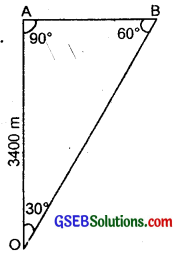Let A be the position of the aircraft at time t = 0. It is just above the observation point O as shown in the figure here. Let after t = 10 sec. the aircraft reaches at point B such that ∠AOB = 30°
OA = 3400 m
Distance covered by the aircraft in 10s = AB
Now in right angled ∆OAB,
$$\frac{AB}{OA}$$ = tan 30° = $$\frac{1}{\sqrt{3}}$$
or AB = $$\frac{OA}{\sqrt{3}}$$ = $$\frac{3400}{1.732}$$ m
If v be the speed of the aircraft, then it is obtained from the formula,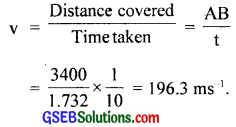Aliter:
OA = 3400 m
In rt. ∠d ∆OAB, tan 15° = $$\frac{AB}{OA}$$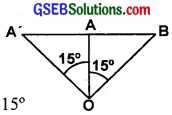or AB = OA tan 15°
= 3400 × 0.2679 = 911 m
As 15° is covered in 5″
∴ v = $$\frac{911}{5}$$ = 182.2 ms-1.